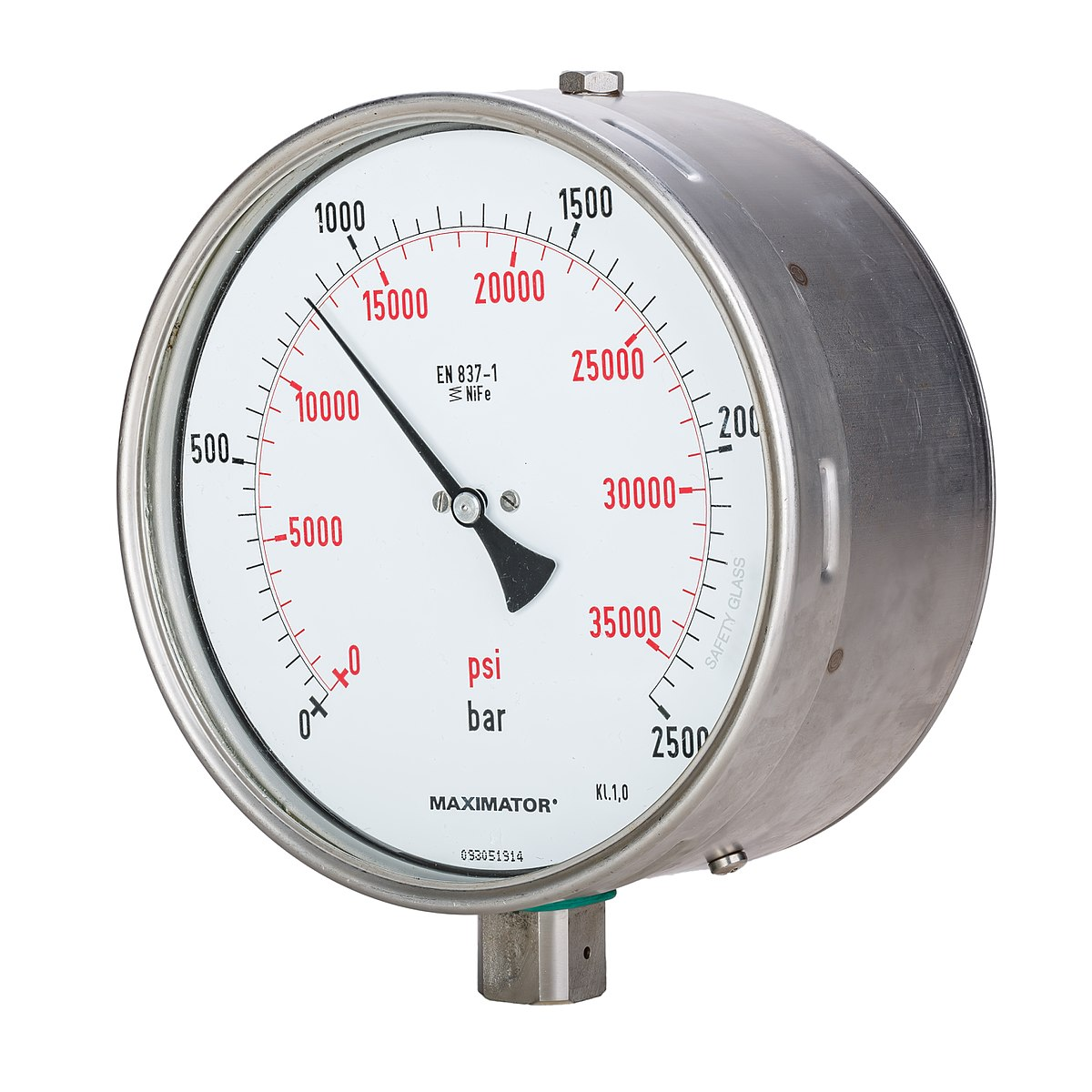Pressure gaugePressure measurement is the analysis of an applied force by a fluid (liquid or gas) on a surface. Pressure is typically measured in units of force per unit of surface area. Many techniques have been developed for the measurement of pressure and vacuum. Instruments used to measure and display pressure in an integral unit are called pressure gauges or vacuum gauges. A manometer is a good example as it uses a column of liquid to both measure and indicate pressure. Likewise the widely used Bourdon gauge is a mechanical device which both measures and indicates and is probably the best known type of gauge. A vacuum gauge is a pressure gauge used to measure pressures lower than the ambient atmospheric pressure, which is set as the zero point, in negative values (e.g.: -15 psi or -760 mmHg equals total vacuum). Most gauges measure pressure relative to atmospheric pressure as the zero point, so this form of reading is simply referred to as "gauge pressure". However, anything greater than total vacuum is technically a form of pressure. For very accurate readings, especially at very low pressures, a gauge that uses total vacuum as the zero point may be used, giving pressure readings in an absolute scale.